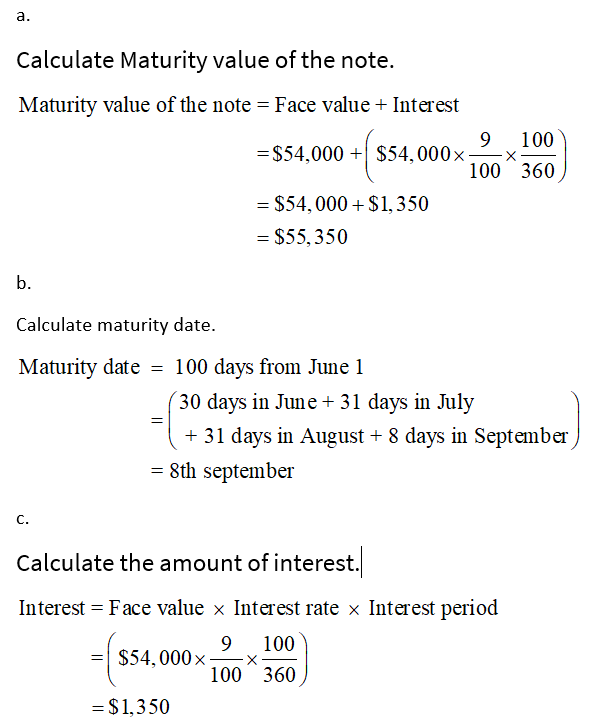# A 100-day note with a face amount of \$54,000 and interest rate of 9% is issued on June 1.Required: 1.Compute the following using a 360-day year:  a. Maturity value of the note\$___________ b. Maturity date___________ c. Interest\$___________2.The note is discounted on July 1. The bank discount rate is 12%. Compute the following: a. Number of days in the discount period___________ b. Discount amount\$___________ c. Proceeds\$___________

Question
19 views

A 100-day note with a face amount of \$54,000 and interest rate of 9% is issued on June 1.

Required:

1.Compute the following using a 360-day year:  a. Maturity value of the note

\$___________

b. Maturity date

___________

c. Interest

\$___________

2.The note is discounted on July 1. The bank discount rate is 12%. Compute the following: a. Number of days in the discount period

___________

b. Discount amount

\$___________

c. Proceeds

\$___________

check_circle

Step 1

1....

### Want to see the full answer?

See Solution

#### Want to see this answer and more?

Solutions are written by subject experts who are available 24/7. Questions are typically answered within 1 hour.*

See Solution
*Response times may vary by subject and question.
Tagged in